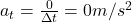## A spaceship negotiates a circular turn of radius 2925 km at a speed of 29960 km/h. (a) What is the magnitude of the angular speed? 0.0028

Question

A spaceship negotiates a circular turn of radius 2925 km at a speed of 29960 km/h. (a) What is the magnitude of the angular speed? 0.0028 Correct: Your answer is correct. rad/s (b) What is the magnitude of the radial acceleration? 23.68 Correct: Your answer is correct. m/s2 (c) What is the magnitude of the tangential acceleration? m/s2

in progress 0
2 months 2021-07-31T19:10:31+00:00 1 Answers 2 views 0

b)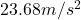c)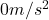Explanation:

a)

When an object is in circular motion, the angular speed of the object is the rate of change of its angular position. In formula, it is given by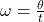where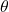is the angular displacement

t is the time interval

The angular speed of an object in circular motion can also be written as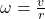(1)

where

v is the linear speed of the object

r is the radius of the orbit

For the spaceship in this problem we have: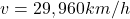is the linear speed, converted into m/s,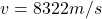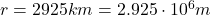is the radius of the orbit

Subsituting into eq(1), we find the angular speed of the spaceship: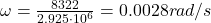b)

When an object is in circular motion, its direction is constantly changing, therefore the object is accelerating; in particular, there is a component of the acceleration acting towards the  centre of the orbit: this is called centripetal acceleration, or radial acceleration.

The magnitude of the radial acceleration is given by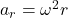where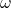is the angular speed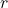is the radius of the orbit

For the spaceship in the problem, we have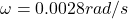is the angular speedis the radius of the orbit

Substittuing into the equation above, we find the radial acceleration: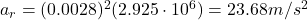c)

When an object is in circular motion, it can also have a component of the acceleration in the direction tangential to its motion: this component is called tangential acceleration.

The tangential acceleration is given by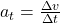where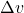is the change in the linear speed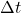is the time interval

In this problem, the spaceship is moving with constant linear speed equal toTherefore, its linear speed is not changing, so the change in linear speed is zero: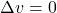And therefore, the tangential acceleration is zero as well: